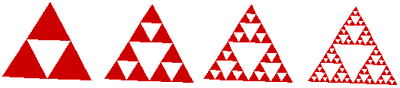# Medial PropertiesThe white triangle on the left is formed by connecting the midpoints of the sides of the red triangle. The second triangle on the left illustrates that smaller white triangles are formed by connecting the midpoints of the sides of the remaining red triangles. The process can continue as long as you want in your mind since drawing all of the small triangles would be nearly impossible after a while!

Find the probability that a point randomly selected in the large triangle will also be in a red triangle for each of the four diagrams.Extension:
Suppose you connect midpoints beyond the fourth illustration for the rest of your life. What is the probability that a point randomly selected in the large triangle will also be in a red region? Explain how you found your solution.